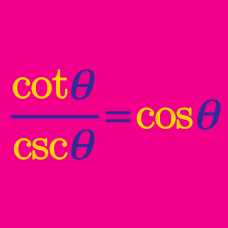Geometry

# Proving Trigonometric Identities

Which of the following expressions is equal to $\left( \sin{\theta} - \tan{\theta} \right) \left( \cos{\theta} - \cot{\theta} \right) ?$

Which of the following expressions is equal to $\tan{\theta} \sin{\theta} + \cos{\theta} ?$

Which of the following expressions is equal to $\tan^2{\theta} + \cot^2{\theta} ?$

Which of the following is equal to $\sin 2x?$

Which of the following expressions is equal to $\tan^2{\theta} - \sin^2{\theta} ?$

×